Next: Operators Up: Fundamental Concepts Previous: Ket Space

# Bra Space

A snack machine inputs coins plus some code entered on a key pad, and (hopefully) outputs a snack. It also does so in a deterministic manner: i.e., the same money plus the same code produces the same snack (or the same error message) time after time. Note that the input and output of the machine have completely different natures. We can imagine building a rather abstract snack machine which inputs ket vectors and outputs complex numbers in a deterministic fashion. Mathematicians call such a machine a functional. Imagine a general functional, labeled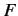, acting on a general ket vector, labeled, and spitting out a general complex number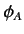. This process is represented mathematically by writing(9)

Let us narrow our focus to those functionals that preserve the linear dependencies of the ket vectors upon which they operate. Not surprisingly, such functionals are termed linear functionals. A general linear functional, labeled, satisfies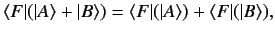(10)

whereand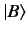are any two kets in a given ket space.

Consider an-dimensional ket space [i.e., a finite-dimensional, or denumerably infinite dimensional (i.e.,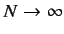), space]. Let the(where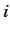runs from 1 to) representindependent ket vectors in this space. A general ket vector can be written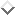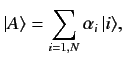(11)

where the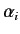are an arbitrary set of complex numbers. The only way that the functionalcan satisfy Equation (10) for all vectors in the ket space is if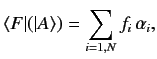(12)

where the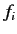are a set of complex numbers relating to the functional.

Let us definebasis functionals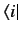which satisfy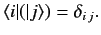(13)

Here, the Kronecker delta symbol is defined such thatif, and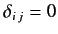otherwise. It follows from the previous three equations that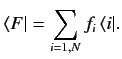(14)

But, this implies that the set of all possible linear functionals acting on an-dimensional ket space is itself an-dimensional vector space. This type of vector space is called a bra space (after Dirac), and its constituent vectors (which are actually functionals of the ket space) are called bra vectors. Note that bra vectors are quite different in nature to ket vectors (hence, these vectors are written in mirror image notation,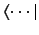and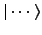, so that they can never be confused). Bra space is an example of what mathematicians call a dual vector space (i.e., it is dual to the original ket space). There is a one to one correspondence between the elements of the ket space and those of the related bra space. So, for every elementof the ket space, there is a corresponding element, which it is also convenient to label, in the bra space. That is,(15)

where DC stands for dual correspondence.

There are an infinite number of ways of setting up the correspondence between vectors in a ket space and those in the related bra space. However, only one of these has any physical significance. (See Section 1.11.) For a general ket vector, specified by Equation (11), the corresponding bra vector is written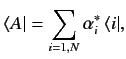(16)

where the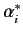are the complex conjugates of the.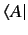is termed the dual vector to. It follows, from the above, that the dual to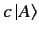is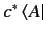, where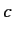is a complex number. More generally,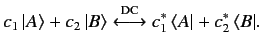(17)

Recall that a bra vector is a functional that acts on a general ket vector, and spits out a complex number. Consider the functional which is dual to the ket vector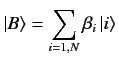(18)

acting on the ket vector. This operation is denoted. Note, however, that we can omit the round brackets without causing any ambiguity, so the operation can also be written. This expression can be further simplified to give. According to Equations (11), (13), (16), and (18),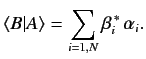(19)

Mathematicians termthe inner product of a bra and a ket.An inner product is (almost) analogous to a scalar product between covariant and contravariant vectors in curvilinear coordinates. It is easily demonstrated that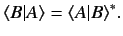(20)

Consider the special case where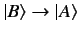. It follows from Equations (19) and (20) thatis a real number, and that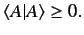(21)

The equality sign only holds ifis the null ket [i.e., if all of theare zero in Equation (11)]. This property of bra and ket vectors is essential for the probabilistic interpretation of quantum mechanics, as will become apparent in Section 1.11.

Two ketsandare said to be orthogonal if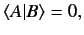(22)

which also implies that.

Given a ket, which is not the null ket, we can define a normalized ket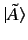, where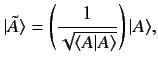(23)

with the property(24)

Here,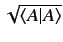is known as the norm or length'' of, and is analogous to the length, or magnitude, of a conventional vector. Becauseandrepresent the same physical state, it makes sense to require that all kets corresponding to physical states have unit norms.

It is possible to define a dual bra space for a ket space of nondenumerably infinite dimensions in much the same manner as that described above. The main differences are that summations over discrete labels become integrations over continuous labels, Kronecker delta symbols become Dirac delta functions, completeness must be assumed (it cannot be proved), and the normalization convention is somewhat different. (See Section 1.15.)Next: Operators Up: Fundamental Concepts Previous: Ket Space
Richard Fitzpatrick 2013-04-08